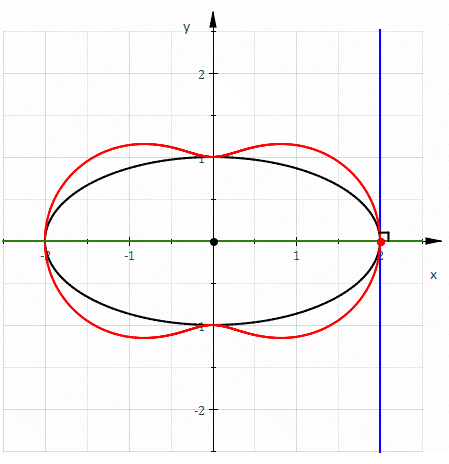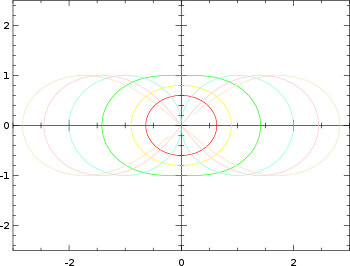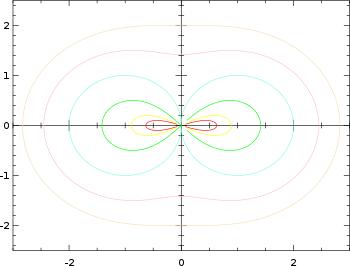# HippopedeHippopede (red) given as the pedal curve of an ellipse (black). The equation of the hippopede is 4x2+y2=(x2+y2)2.

In geometry, a hippopede (from Ancient Greek ἱπποπέδη, "horse fetter") is a plane curve determined by an equation of the form,

where it is assumed that c>0 and c>d since the remaining cases either reduce to a single point or can be put into the given form with a rotation. Hippopedes are bicircular rational algebraic curves of degree 4 and symmetric with respect to both the x and y axes.

## Special cases

When d>0 the curve has an oval form and is often known as an oval of Booth, and when d<0 the curve resembles a sideways figure eight, or lemniscate, and is often known as a lemniscate of Booth, after 19th-century mathematician James Booth who studied them. Hippopedes were also investigated by Proclus (for whom they are sometimes called Hippopedes of Proclus) and Eudoxus. For d = c, the hippopede corresponds to the lemniscate of Bernoulli.

## Definition as spiric sectionsHippopedes with a = 1, b = 0.1, 0.2, 0.5, 1.0, 1.5, and 2.0.Hippopedes with b = 1, a = 0.1, 0.2, 0.5, 1.0, 1.5, and 2.0.

Hippopedes can be defined as the curve formed by the intersection of a torus and a plane, where the plane is parallel to the axis of the torus and tangent to it on the interior circle. Thus it is a spiric section which in turn is a type of toric section.

If a circle with radius a is rotated about an axis at distance b from its center, then the equation of the resulting hippopede in polar coordinates.

Note that when a>b the torus intersects itself, so it does not resemble the usual picture of a torus.